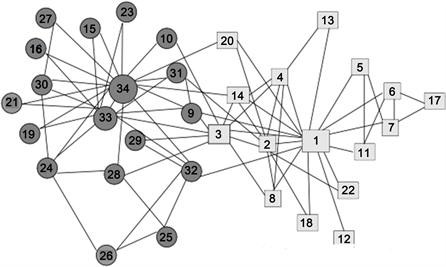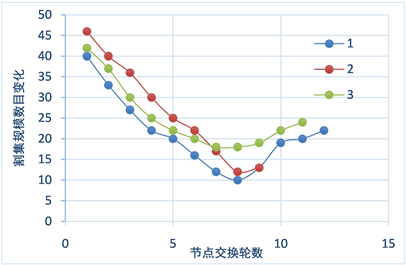﻿ Kernighan-Lin算法的改进对图划分问题的研究 Research on the Improvement of Kernighan-Lin Algorithm for Graph Partitioning

Computer Science and Application
Vol. 09  No. 05 ( 2019 ), Article ID: 30120 , 6 pages
10.12677/CSA.2019.95095

Research on the Improvement of Kernighan-Lin Algorithm for Graph Partitioning

Yong Yu, Shilin Kan, Na Zhao, Yongjun Luo, Jie Gu, Chenming Song

Key Laboratory in Software Engineering of Yunnan Province, School of Software, Yunnan University, Kunming YunnanReceived: Apr. 24th, 2019; accepted: May 2nd, 2019; published: May 9th, 2019ABSTRACT

With the development of Internet technology and the advent of the era of big data, the community division of complex networks has become a research hotspot. Among them, the large graph iterative calculation is the focus of its research, and the reduction of the communication edge between sub-graphs is to improve the computing capability. Kernighan-Lin algorithm is one of the simplest and most well-known heuristic algorithms in graph partitioning problem. Because traditional Kernighan-Lin algorithm is difficult to improve its execution efficiency in graph partitioning problem, this paper is based on the idea and graph of traditional algorithm. After the research of partitioning problem, an improved algorithm for Kernighan-Lin algorithm is proposed. Finally, using the karate club dataset as experimental data, the experimental results demonstrate the effectiveness and feasibility of the improved algorithm.

Keywords:Kernighan-Lin Algorithm, Graph Partitioning, Social Network

Kernighan-Lin算法的改进对图 划分问题的研究1. 引言

2. 传统Kernighan-Lin算法及分析

Kernighan-Lin算法是由Brian Kernighan和Shen Lin在1970年提出的，是图划分问题中最简单、最知名的启发式算法之一  。核心思想是将n个图节点任意划分成指定规模的两个群组，对于任何由分属不同群组的节点i和节点j组成的顶点对(i, j)，交换i和j的位置，并计算交换前后两个群组之间割集规模的变化量。在所有(i, j)对中找到使割集规模减小最多的顶点对，或者若没有使割集规模减小的顶点对，则找到使割集规模增加最小的顶点对，然后交换这个两个顶点。在交换的过程中，两个群组的节点数量都不变，因为一个节点离开某个群组时另一个节点加入该群组。因此，算法能够满足群组的规模与指定规模保持一致。然后重复上述过程，但一个重要的约束条件是群组中的每个节点只能移动一次。一旦某个节点和另一个节点交换过，那它将不会再次被交换(至少不会在本轮算法中再次被交换)。因此，在算法第二步中，考虑除第一步中交换过的两个节点之外的所有顶点对。接着算法继续运行，每一步都交换最大程度减少或最小程度增加群组之间边数的顶点对，直到没有可以交换的顶点对，此时本轮算法停止。若群组规模不相等，那么在较大规模群组中会有未被交换过的节点，其数量等于群组规模的差值。

Kernighan-Lin算法可描述为下面的步骤：

Step1：给定N个节点的图，随机划分图节点到两个指定规模大小的 ${n}_{1},{n}_{2}$ 群组；

Step2：分别从 ${n}_{1},{n}_{2}$ 群组中选择节点i和节点j组成顶点对(i, j)，交换i和j的位置，并计算交换前后群组之间割集规模的变化量并记录该变化量P；

Step3：重复上步骤，已交换过的节点不再参与；

Step4：直到没有可以交换的顶点对，算法结束。

3. 改进算法及算法分析

3.1. 改进算法描述

1) 按照要求随机分成两个群组V1和群组V2，其中 $|{V}_{1}|={n}_{1},|{V}_{2}|={n}_{2},V={V}_{1}\cup {V}_{2}$ ，对群组V1和群组V2中的每个节点及其它对于群组V1和群组V2的度可以用一个三元组 $\left(v,{\Gamma }_{1},{\Gamma }_{2}\right)$ 来表示，V是对应的节点名， ${\Gamma }_{1}$ 表示节点V在群组V1中的度， ${\Gamma }_{2}$ 表示节点V在群组V2中的度，即 ${\Gamma }_{1}\left(v\right)=|\left\{{v}^{\prime }\in {V}_{1}|\left(v,{v}^{\prime }\right)\in E\right\}|$ 表示节点V与群组V1中的其它节点之间的连接边数； ${\Gamma }_{2}\left(v\right)=|\left\{{v}^{\prime }\in {V}_{2}|\left(v,{v}^{\prime }\right)\in E\right\}|$ 表示节点V与群组V2中的其它节点之间的连接边数；且对于任意的节点V都有 ${\Gamma }_{1}\left(v\right)+{\Gamma }_{2}\left(v\right)=\Gamma \left(v\right)$

2) 当图 $G=\left(V,E\right)$ 被划分为两个群组V1和群组V2后，对应的割集中边的数目为 $|CS|=\underset{{v}_{i}\in {V}_{1}}{\sum }{\Gamma }_{2}\left({v}_{i}\right)+\underset{{v}_{i}\in {V}_{2}}{\sum }{\Gamma }_{1}\left({v}_{i}\right)$

3) 目标是使得群组内的节点之间的边数最大，而群组之间的边数最小，即：

$\mathrm{max}\left(\underset{{v}_{i}\in {V}_{1}}{\sum }{\Gamma }_{1}\left({v}_{i}\right)+\underset{{v}_{i}\in {V}_{2}}{\sum }{\Gamma }_{2}\left({v}_{i}\right)\right)$ 并且 $\mathrm{min}\left(\underset{{v}_{i}\in {V}_{1}}{\sum }{\Gamma }_{2}\left({v}_{i}\right)+\underset{{v}_{i}\in {V}_{2}}{\sum }{\Gamma }_{1}\left({v}_{i}\right)\right)$ ，但是由于 $\left(\underset{{v}_{i}\in {V}_{1}}{\sum }{\Gamma }_{1}\left({v}_{i}\right)+\underset{{v}_{i}\in {V}_{2}}{\sum }{\Gamma }_{2}\left({v}_{i}\right)\right)+\left(\underset{{v}_{i}\in {V}_{1}}{\sum }{\Gamma }_{2}\left({v}_{i}\right)+\underset{{v}_{i}\in {V}_{2}}{\sum }{\Gamma }_{1}\left({v}_{i}\right)\right)=2m$ 是个常数(m为图的总边数)，因此函数 $\mathrm{max}\left(\underset{{v}_{i}\in {V}_{1}}{\sum }{\Gamma }_{1}\left({v}_{i}\right)+\underset{{v}_{i}\in {V}_{2}}{\sum }{\Gamma }_{2}\left({v}_{i}\right)\right)$$\mathrm{min}\left(\underset{{v}_{i}\in {V}_{1}}{\sum }{\Gamma }_{2}\left({v}_{i}\right)+\underset{{v}_{i}\in {V}_{2}}{\sum }{\Gamma }_{1}\left({v}_{i}\right)\right)$ 等价的，故可以选择 $\mathrm{min}\left(\underset{{v}_{i}\in {V}_{1}}{\sum }{\Gamma }_{2}\left({v}_{i}\right)+\underset{{v}_{i}\in {V}_{2}}{\sum }{\Gamma }_{1}\left({v}_{i}\right)\right)$ 即割集边数最小化为目标函数；

4) 分别选择每个群组中外边数减去内边数最大的两个节点组成2 × 2个顶点对；

5) 选择四种交换中割集规模减少最大或者割集规模增加最少的作为最终的交换顶点对；即可能的四次变换中 $\left(\underset{{v}_{i}\in {V}_{1}}{\sum }{\Gamma }_{2}\left({v}_{i}\right)+\underset{{v}_{i}\in {V}_{2}}{\sum }{\Gamma }_{1}\left({v}_{i}\right)\right)$ 减少最大或者增加最少；

6) 交换过的节点不再参与，直到无法在某个群组中找到可交换的两个节点，算法结束。

3.2. Kernighan-Lin改进算法节点度变化分析

1) 对于节点 ${v}_{i}$${v}_{j}$$〈{v}_{i},{\Gamma }_{1}\left({v}_{i}\right),{\Gamma }_{2}\left({v}_{i}\right)〉$$〈{v}_{j},{\Gamma }_{1}\left({v}_{j}\right),{\Gamma }_{2}\left({v}_{j}\right)〉$ 换成 $〈{v}_{i},{\Gamma }_{2}\left({v}_{i}\right),{\Gamma }_{1}\left({v}_{i}\right)〉$$〈{v}_{j},{\Gamma }_{2}\left({v}_{j}\right),{\Gamma }_{1}\left({v}_{j}\right)〉$

2) 对于 ${V}_{1}$${V}_{2}$ 中其他节点 ${v}_{s}$ 可以分为如下几种情况：

$〈{v}_{s},{v}_{i}〉\in E\wedge 〈{v}_{s},{v}_{j}〉\in E$ ，则节点 ${v}_{s}$ 的度情况  保持不变；

$〈{v}_{s},{v}_{i}〉\in E\wedge 〈{v}_{s},{v}_{j}〉\notin E$ ，则节点 ${v}_{s}$ 的度情况 $〈{v}_{s},{\Gamma }_{1}\left({v}_{s}\right),{\Gamma }_{2}\left({v}_{s}\right)〉$ 变为 $〈{v}_{s},{\Gamma }_{1}\left({v}_{s}\right)-1,{\Gamma }_{2}\left({v}_{s}\right)+1〉$

$〈{v}_{s},{v}_{i}〉\notin E\wedge 〈{v}_{s},{v}_{j}〉\in E$ ，则节点 ${v}_{s}$ 的度情况 $〈{v}_{s},{\Gamma }_{1}\left({v}_{s}\right),{\Gamma }_{2}\left({v}_{s}\right)〉$ 变为 $〈{v}_{s},{\Gamma }_{1}\left({v}_{s}\right)+1,{\Gamma }_{2}\left({v}_{s}\right)-1〉$ .

3.3. 改进算法分析

$O\left(n\right)$ ，每轮选取之后的迭代次数为4，总的轮数为节点选取次数。因此，整个算法时间复杂度约为 $O\left({n}^{2}\right)$ .而在传统的算法中，其时间复杂度大于 $O\left({n}^{2}\right)$ 。改进算法一方面从时间复杂度有所突破，另一方面其执行效率也明显提高。

4. 实验

4.1. 实验方案与环境

Zachary是通过对一个美国大学空手道俱乐部  进行观测而构建出的一个社交网络关系图  。如图1所示，图包含34个节点和78条边，其中个体表示俱乐部中的成员，而边表示成员之间存在的友谊关系，空手道俱乐部网络图已经成为图划分中的一个经典问题。因此本节选择Zachary空手道俱乐部网络图的数据集为实验数据，使得该实验更加具有合理性和充分性。Figure 1. Karate club network

4.2. 实验结果与分析Figure 2. Changes between networks

5. 结论

Research on the Improvement of Kernighan-Lin Algorithm for Graph Partitioning[J]. 计算机科学与应用, 2019, 09(05): 849-854. https://doi.org/10.12677/CSA.2019.95095

1. 1. 张军, 熊枫, 张丹. 图像隐写分析技术综述[J]. 计算机工程, 2013, 39(4): 165-168.

2. 2. 李琪, 钟将, 李雪. 基于启发策略的动态平衡图划分算法[J]. 计算机研究与发展, 2017(12): 2834-2840.

3. 3. 郑丽丽. 带权图的k划分算法研究[D]: [硕士学位论文]. 天津: 天津工业大学, 2013.

4. 4. 许金凤, 董一鸿, 王诗懿, 等. LGP-SA: 分布式环境下基于模拟退火的大规模图划分算法[J]. 电信科学, 2016, 32(2): 83-91.

5. 5. 姜火文, 曾国荪, 胡克坤. 一种遗传算法实现的图聚类匿名隐私保护方法[J]. 计算机研究与发展, 2016, 53(10): 2354-2364.

6. 6. Saab, Y.G. (2004) An Effective Multilevel Algorithm for Bisecting Graphs and Hypergraphs. IEEE Transactions on Computers, 53, 641-652. https://doi.org/10.1109/TC.2004.3

7. 7. Benlic, U. and Hao, J.K. (2011) A Mul-tilevel Memetic Approach for Improving Graph k-Partitions. IEEE Transactions on Evolutionary Computation, 15, 624-642. https://doi.org/10.1109/TEVC.2011.2136346

8. 8. 曾华, 崔文, 付连宁, 等. Lin-Kernighan算法初始解的启发式构造策略[J]. 山东大学学报(工学版), 2012, 42(2): 30-35.

9. 9. Pellegrini, F. and Roman, J. (1996) Scotch: A Software Package for Static Mapping by Dual Recursive Bi-Partitioning of Process and Architecture Graphs. In: Proceedings of HPCN Europe, Springer, Berlin, 493-498. https://doi.org/10.1007/3-540-61142-8_588

10. 10. Simon, H.D. (1991) Partitioning of Unstructured Problems for Parallel Pro-cessing. Computing Systems in Engineering, 2, 135-148. https://doi.org/10.1016/0956-0521(91)90014-V

11. 11. Karypis, G. and Kumar, V. (1998) Multilevel K-Way Partitioning Scheme for Irregular Graphs. Journal of Parallel and Distributed Computing, 48, 96-129. https://doi.org/10.1006/jpdc.1997.1404

12. 12. Grady, L. and Schwartz, E.L. (2006) Isoperimetric Graph Partitioning for Image Segmentation. IEEE Transactions on Pattern Analysis & Machine Intelligence, 28, 469-475. https://doi.org/10.1109/TPAMI.2006.57

13. 13. Kernighan, B.W. and Lin, S.A. (1970) Efficient Heuristic Procedure for Partitioning Graphs. Bell System Technical Journal, 49, 291-307. https://doi.org/10.1002/j.1538-7305.1970.tb01770.x

14. 14. 李孔文, 顾庆, 张尧, 等. 一种基于聚集系数的局部社团划分算法[J]. 计算机科学, 2010, 37(7): 46-49.

15. 15. 杨艳萍. 基于双向加权图的社交网络用户可信度算法研究[J]. 信息网络安全, 2017, 17(7): 40-44.